# DCM mini tutorial #1 – Smooth Push

I made this DCM(DataChannel Modifier) while ago to help my friend’s shot. I thought that it might be worth as my first DCM mini tutorial. So, here we go!

Let’s see the following picture. This is the famous Stanford Bunny model. Left is the original shape. The right is 3dsMax Push modifier. As you can see, when you push a lot the faces start to overlap. The middle is the result of “Smooth Push” which is a simple DCM setup. As you can see It pushed it, but it pushes more gently(?).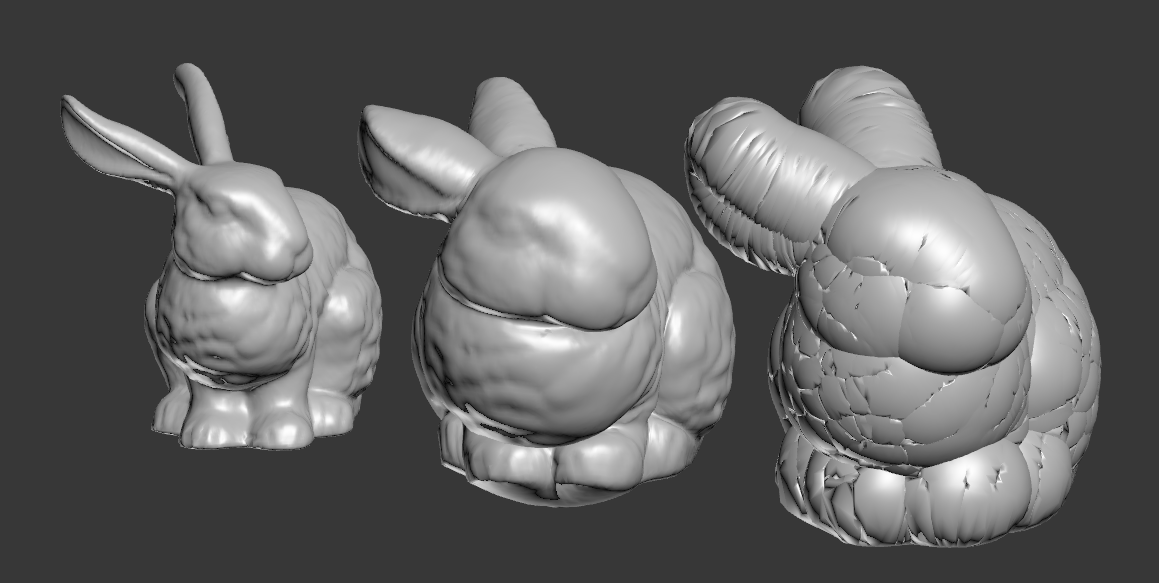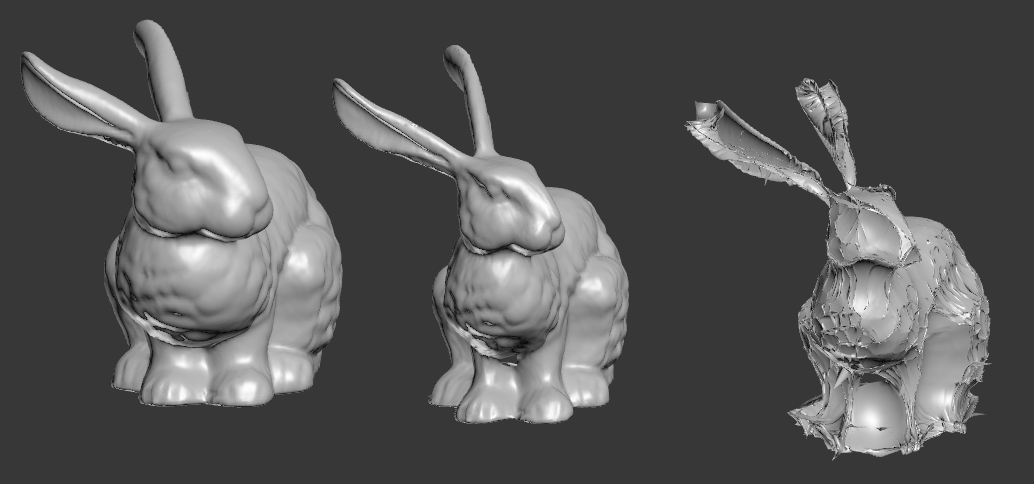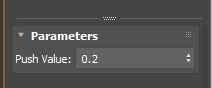How Push modifier works is really really simple. It moves each verts along the its normal by the given amount. As you can see, Push modifier only has one parameter which is the distance the verts are moved along normals.

Becase the verts move along the normal, verts will meet each other if you have concave shape. To prevent that, I simple smooth or blurred or relaxed the normals and used that. Let’s see how that translate to DCM setup.

First, we need to get vertex normal for each verts.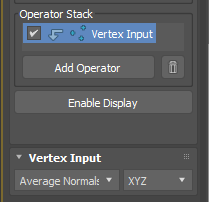3. Choose “Average Normals”.

As an Input operator, Vertex Input allow you to grab various data from each verts. The 3 dot icon in front of operator name indicates, you are processing vertex data.

Next, Add “Smooth” operator from Process operators. It has 2 values, Iteration and Amount just like Relax modifier. The bigger Iteration and Amount is, the the smoother result you will get. This operator will make more gentle normals by averaging normals with neighboring normals just like blurring an image.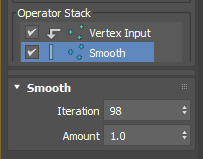Now we need to have a way to control amount of Push. This is simple. Let’s add “Scale” operator from Process operators. This operator multiplies the given value to float or vector. If the value is 1.0. The size of normal doesn’t change. If the value is 1.0, you ar making normal bigger. If the value is less than 1.0, you are making normal smaller. This is exactly what “Push value” in Push modifier does.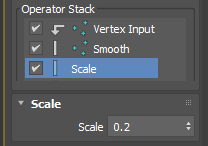What we have now in the current Data Channel is the offset vector of the each vertex. So, you need to add these vectors to the original vertex position.

1. Add Vertex Output operator from Output operators.
2. Choose “Position” as output channel.
3. Change “Selection Method” from “Replace” to “Add”, which means you will add the current channel data to the existing vertex position data.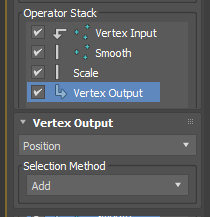Dadah! You have a Smooth Push! Easy, right?